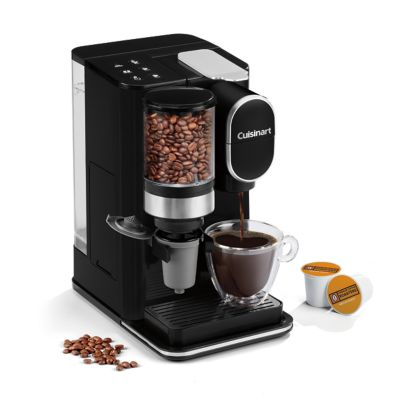# Coffee Makers

## Coffee Makers

Showing 1 - 48 of 183 products
Earn 3x points
Earn 3x points
Earn 3x points
Earn 3x points
Cuisinart
194 reviews
\$149.99

Online Only

In stock for standard shippingEarn 3x points
Earn 3x points
Earn 3x points
Earn 3x points
Earn 3x points
Earn 3x points
Earn 3x points
Earn 3x points
Earn 3x points
Earn 3x points
Earn 3x points
Earn 3x points
Earn 3x points
Earn 3x points
Earn 3x points
Earn 3x points
Earn 3x points
Earn 3x points
Earn 3x points
Earn 3x points
Earn 3x points
Earn 3x points
Earn 3x points
Earn 3x points
Earn 3x points
Earn 3x points
Earn 3x points
Earn 3x points
Earn 3x points
Earn 3x points
Earn 3x points
Earn 3x points